# resample

Resample uniform or nonuniform data to new fixed rate

## Syntax

y = resample(x,p,q)
y = resample(x,p,q,n)
y = resample(x,p,q,n,beta)
y = resample(x,p,q,b)
[y,b] = resample(x,p,q,___)
y = resample(x,tx)
y = resample(x,tx,fs)
y = resample(x,tx,fs,p,q)
y = resample(x,tx,___,method)
[y,ty] = resample(x,tx,___)
[y,ty,b] = resample(x,tx,___)

## Description

example

y = resample(x,p,q) resamples the input sequence, x, at p/q times the original sample rate. If x is a matrix, then resample treats each column of x as an independent channel. resample applies an antialiasing FIR lowpass filter to x and compensates for the delay introduced by the filter.
y = resample(x,p,q,n) uses an antialiasing filter of order 2 × n × max(p,q).

example

y = resample(x,p,q,n,beta) specifies the shape parameter of the Kaiser window used to design the lowpass filter.
y = resample(x,p,q,b) filters x using the filter coefficients specified in b.

example

[y,b] = resample(x,p,q,___) also returns the coefficients of the filter applied to x during the resampling.
y = resample(x,tx) resamples the values, x, of a signal sampled at the instants specified in vector tx. The function interpolates x linearly onto a vector of uniformly spaced instants with the same endpoints and number of samples as tx. NaNs are treated as missing data and are ignored.
y = resample(x,tx,fs) uses a polyphase antialiasing filter to resample the signal at the uniform sample rate specified in fs.
y = resample(x,tx,fs,p,q) interpolates the input signal to an intermediate uniform grid with a sample spacing of (p/q)/fs. The function then filters the result to upsample it by p and downsample it by q, resulting in a final sample rate of fs. For best results, ensure that fs × q/p is at least twice as large as the highest frequency component of x.

example

y = resample(x,tx,___,method) specifies the interpolation method along with any of the arguments from previous syntaxes in this group. The interpolation method can be 'linear', 'pchip', or 'spline'.Note:   If x is not slowly varying, consider using interp1 with the 'pchip' interpolation method.
[y,ty] = resample(x,tx,___) returns in ty the instants that correspond to the resampled signal.
[y,ty,b] = resample(x,tx,___) returns in b the coefficients of the antialiasing filter.

## Examples

collapse all

Resample a simple linear sequence at 3/2 the original rate of 10 Hz. Plot the original and resampled signals on the same figure.

fs = 10; t1 = 0:1/fs:1; x = t1; y = resample(x,3,2); t2 = (0:(length(y)-1))*2/(3*fs); plot(t1,x,'*',t2,y,'o') xlabel('Time (s)') ylabel('Signal') legend('Original','Resampled', ... 'Location','NorthWest')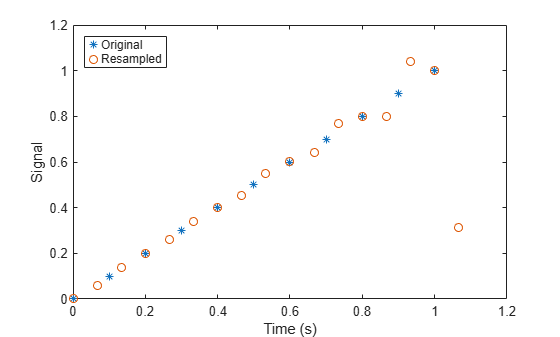When filtering, resample assumes that the input sequence, x, is zero before and after the samples it is given. Large deviations from zero at the endpoints of x can result in unexpected values for y.

Show these deviations by resampling a triangular sequence and a vertically shifted version of the sequence with nonzero endpoints.

x = [1:10 9:-1:1; 10:-1:1 2:10]'; y = resample(x,3,2); subplot(2,1,1) plot(1:19,x(:,1),'*',(0:28)*2/3 + 1,y(:,1),'o') title('Edge Effects Not Noticeable') legend('Original','Resampled', ... 'Location','South') subplot(2,1,2) plot(1:19,x(:,2),'*',(0:28)*2/3 + 1,y(:,2),'o') title('Edge Effects Noticeable') legend('Original','Resampled', ... 'Location','North')Construct a sinusoidal signal. Specify a sample rate such that 16 samples correspond to exactly one signal period. Draw a stem plot of the signal. Overlay a stairstep graph for sample-and-hold visualization.

fs = 16; t = 0:1/fs:1-1/fs; x = 0.75*sin(2*pi*t); stem(t,x) hold on stairs(t,x) hold off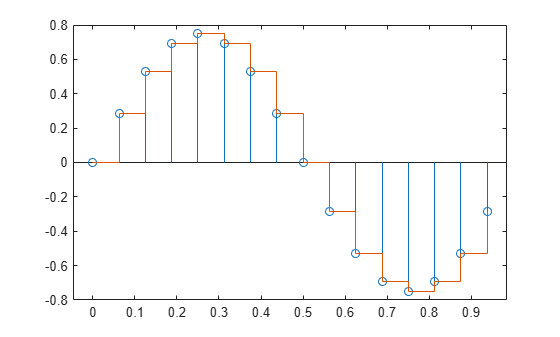Use resample to upsample the signal by a factor of four. Use the default settings. Plot the result alongside the original signal.

ups = 4; dns = 1; fu = fs*ups; tu = 0:1/fu:1-1/fu; y = resample(x,ups,dns); stem(tu,y) hold on stairs(t,x) hold off legend('Resampled','Original')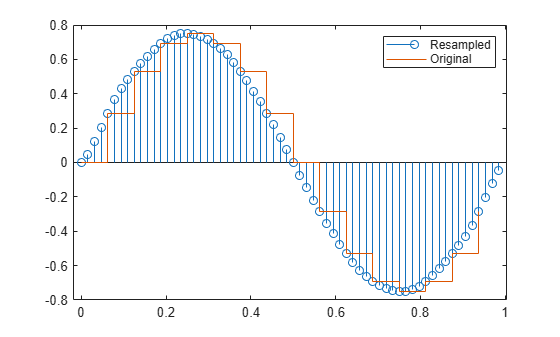Repeat the calculation. Specify n = 1 so that the antialiasing filter is of order $2×1×4=8$. Specify a shape parameter $\beta =0$ for the Kaiser window. Output the filter as well as the resampled signal.

n = 1; beta = 0; [y,b] = resample(x,ups,dns,n,beta); fo = filtord(b)
fo = 8 
stem(tu,y) hold on stairs(t,x,'--') hold off legend('n = 1, \beta = 0')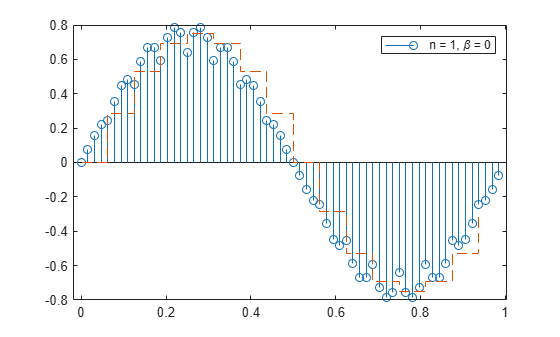The resampled signal shows aliasing effects that result from the relatively wide mainlobe and low sidelobe attenuation of the window.

Increase n to 5 and leave $\beta =0$. Verify that the filter is of order 40. Plot the resampled signal.

n = 5; [y,b] = resample(x,ups,dns,n,beta); fo = filtord(b)
fo = 40 
stem(tu,y) hold on stairs(t,x,'--') hold off legend('n = 5, \beta = 0')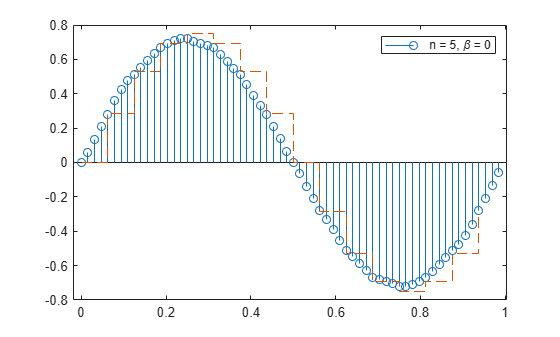The longer window has a narrower mainlobe and attenuates aliasing effects better. It also attenuates the signal.

Leave the filter order at $2×5×4=40$ and increase the shape parameter to $\beta =20$.

beta = 20; y = resample(x,ups,dns,n,beta); stem(tu,y) hold on stairs(t,x,'--') hold off legend('n = 5, \beta = 20')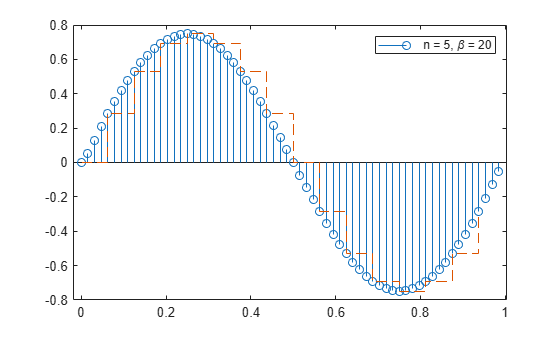The high sidelobe attenuation results in good resampling.

Decrease the filter order back to $2×1×4=8$ and leave $\beta =20$.

n = 1; [y,b] = resample(x,ups,dns,n,beta); stem(tu,y) hold on stairs(t,x,'--') hold off legend('n = 1, \beta = 20')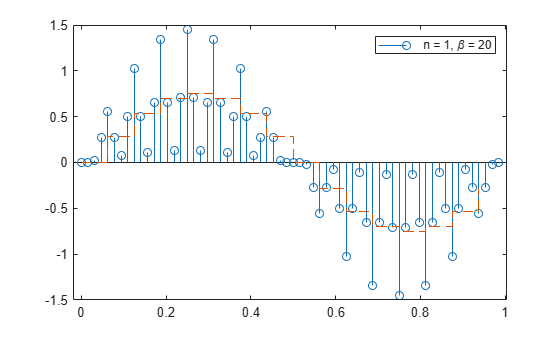The wider mainlobe generates considerable artifacts upon resampling.

Generate 60 samples of a sinusoid and resample it at 3/2 the original rate. Display the original and resampled signals.

tx = 0:6:360-3; x = sin(2*pi*tx/120); ty = 0:4:360-2; [y,by] = resample(x,3,2); plot(tx,x,'+-',ty,y,'o:') legend('original','resampled')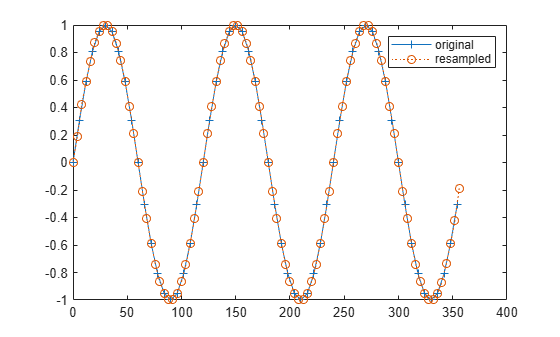Plot the frequency response of the anti-aliasing filter.

freqz(by)Resample the signal at 2/3 the original rate. Display the original signal and its resampling.

tz = 0:9:360-9; [z,bz] = resample(x,2,3); plot(tx,x,'+-',tz,z,'o:') legend('original','resampled')Plot the impulse response of the new lowpass filter.

impz(bz)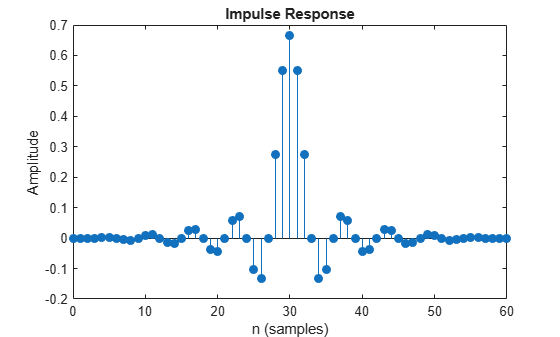Use the data recorded by Galileo Galilei in 1610 to determine the orbital period of Callisto, the outermost of Jupiter's four largest satellites.

Galileo observed the satellites' motion for six weeks, starting on 15 January. The observations have several gaps because Jupiter was not visible on cloudy nights. Generate a datetime array of observation times.

t = [0 2 3 7 8 9 10 11 12 17 18 19 20 24 25 26 27 28 29 31 32 33 35 37 ... 41 42 43 44 45]'+1; yg = [10.5 11.5 10.5 -5.5 -10.0 -12.0 -11.5 -12.0 -7.5 8.5 12.5 12.5 ... 10.5 -6.0 -11.5 -12.5 -12.5 -10.5 -6.5 2.0 8.5 10.5 13.5 10.5 -8.5 ... -10.5 -10.5 -10.0 -8.0]'; obsv = datetime(1610,1,15+t);

Resample the data onto a regular grid using a sample rate of one observation per day. Use a moderate upsampling factor of 3 to avoid overfitting.

fs = 1; [y,ty] = resample(yg,t,fs,3,1);

Plot the data and the resampled signal.

plot(t,yg,'o',ty,y,'.-') xlabel('Day')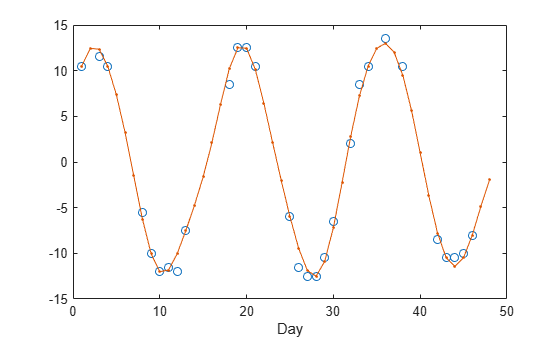Repeat the procedure using spline interpolation and displaying the observation dates. Express the sample rate in inverse days.

fs = 1/86400; [ys,tys] = resample(yg,obsv,fs,3,1,'spline'); plot(t,yg,'o') hold on plot(ys,'.-') hold off ax = gca; ax.XTick = t(1:9:end); ax.XTickLabel = char(obsv(1:9:end));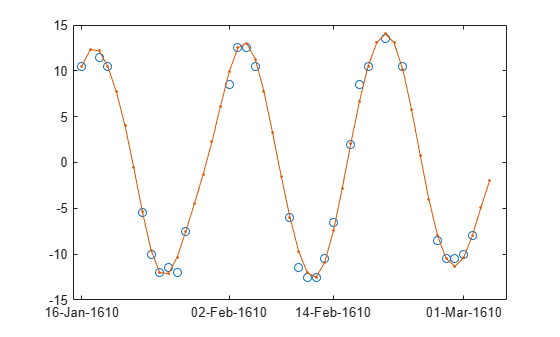Compute the periodogram power spectrum estimate of the uniformly spaced, linearly interpolated data. Choose a DFT length of 1024. The signal peaks at the inverse of the orbital period.

[pxx,f] = periodogram(ys,[],1024,1,'power'); [pk,i0] = max(pxx); f0 = f(i0); T0 = 1/f0
T0 = 16.7869 
plot(f,pxx,f0,pk,'o') xlabel('Frequency (day^{-1})')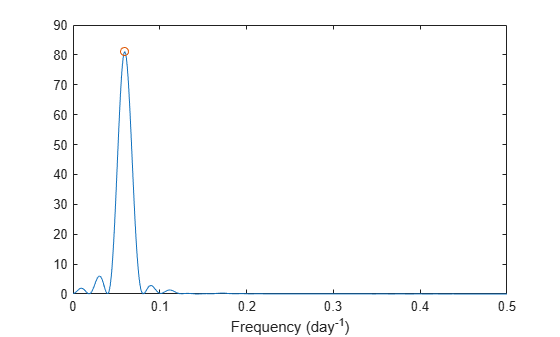## Input Arguments

collapse all

Input signal, specified as a vector or matrix. If x is a matrix, then its columns are treated as independent channels. x can contain NaNs. NaNs are treated as missing data and are excluded from the resampling.

Example: cos(pi/4*(0:159))+randn(1,160) is a single-channel row-vector signal.

Example: cos(pi./[4;2]*(0:159))'+randn(160,2) is a two-channel signal.

Data Types: double

Resampling factors, specified as positive integers.

Data Types: double

Neighbor term number, specified as a positive integer. If n = 0, resample performs nearest-neighbor interpolation. The length of the antialiasing FIR filter is proportional to n. Larger values of n provide better accuracy at the expense of more computation time.

Data Types: double

Shape parameter of Kaiser window, specified as a positive real scalar. Increasing beta widens the mainlobe of the window used to design the antialiasing filter and decreases the amplitude of the window’s sidelobes.

Data Types: double

FIR filter coefficients, specified as a vector. By default, resample designs the filter using firls with a Kaiser window. When compensating for the delay, resample assumes b has odd length and linear phase.

Example: fir1(4,0.5) specifies a 4th-order lowpass filter with normalized cutoff frequency 0.5π rad/sample.

Data Types: double

Time instants, specified as a nonnegative real vector or a datetime array. tx must increase monotonically but need not be uniformly spaced. tx can contain NaNs or NaTs. These values are treated as missing data and excluded from the resampling.

Data Types: double | datetime

Sample rate, specified as a positive scalar. The sample rate is the number of samples per unit time. If the unit of time is seconds, then the sample rate is in Hz.

Data Types: double

Interpolation method, specified as one of 'linear', 'pchip', or 'spline':

• 'linear' — Linear interpolation.

• 'pchip' — Shape-preserving piecewise cubic interpolation.

• 'spline' — Spline interpolation using not-a-knot end conditions.

See the interp1 reference page for more information.

## Output Arguments

collapse all

Resampled signal, returned as a vector or matrix. If x is a signal of length N and you specify p and q, then y is of length ⌈N × p/q⌉.

FIR filter coefficients, returned as a vector.

Output instants, returned as a nonnegative real vector.

## Algorithms

resample performs an FIR design using firls, normalizes the result to account for the processing gain of the window, and then implements a rate change using upfirdn.